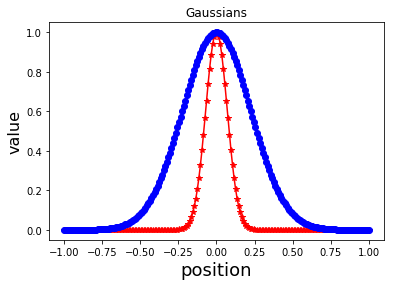# Easy Python¶

Assistant Professor of Chemical Engineering
University of Utah

This is an easy guide for learning Python. In my opinion, you don't need more than this guide to get started with Python. Once you get familiar with the syntax you can dig deeper on your own.

In fact, my attitude towards programming consists of the following two steps:

1. Articulate what you want to accomplish (e.g. I need to sort this array)
2. google it! (e.g. how to sort an array in Python?)

As an engineer, programming languages are fundamental tools in my arsenal to tackle research problems. But they are only a means to an end. Therefore, I am happy to learn programming by mimicking what other professional programmers and software developers do. That's why google is your best friend.

A key reference used for this guide is: Numerical Methods in Engineering with Python 3 by Jaan Kiusalaas.

# Python: Anyone Can Code!¶Python is a modern programming language that is very easy to use. Python promotes productivity and a clear focus on accomplishing the tasks at hand.

In an age of extreme technology and unsurpassed information exchange, it is unacceptable for a modern engineer to NOT know a programming language. Python stands among the easiest and most enjoyable programming languages out there.

If there's ever an example of why someone needs to learn programming, here it is:

In :
from IPython.display import YouTubeVideo

Out:

Back in the 60s, when our nation needed it the most... It was programming that saved the day! (Thanks to Dr. Sean Smith for the suggestion to include a clip from the movie Hidden Figures)

In :
from IPython.display import IFrame
IFrame('https://trinket.io/embed/python3/f144398a16',width="100%",height=300)

Out:

Here's why you should learn Python:

1. Because your future will likely depend on it. Programming job trends rank python among the top. Moreover, coding Dojo places python Numero Uno.
2. Python is FREE!
3. It is very easy to get started programming with Python
4. Python is very forgiving
5. There is significant community support around Python
6. You can even use Python in a web browser!

## Obtaining Python: (Can't you just Google it?)¶

My favorite way of obtaining python is to download the Anaconda distribution: http://www.anaconda.com. Once you install it, Python mysteriously resides on your computer.

## Executing Python Code¶

There are a few ways you can run Python:

1. Write your code in a text file with the extension py. Then from the terminal type python myPythonCode.py
2. Use one of the editors that ship with python. Anaconda ships with Spyder. Another famous editor is called PyCharm . Using these editors (actually Integrated Development Environments, IDEs), you can write code and execute it on the spot.
3. Use Jupyter Notebook. This my favorite approach as it allows you to write Python code from within your browser and mix it with text and equation. Simply open up a terminal (command prompt on windows) and type jupyter notebook.

Here's a Markdown cheat sheet - the language used to write text in Jupyter Notebooks.

# Core Python¶

Python has a bunch of key core functionality including the ability to define variables, strings, lists and other types. Let's get started:

## Variables¶

Variables, just like any programming language, can be declared and assigned on the spot

In :
myVar = 33   # my var is an integer in this case
print(myVar) # this will print the value of myVar

33

In :
b = 3.0 * myVar # b is now floating point variable
print(b)

99.0


Note that Python automatically detects the data type of a variable. This is called dynamic typing.

### Strings¶

Strings are a special type of variable that represent text. Simply use single (or double) quotations to define a string variable.

In :
str1 = 'This is my first string variable.'
print(str1)

This is my first string variable.

In :
str2 = "this is also another string"
print(str2)

this is also another string


You can concatenate strings together:

In :
str3 = str1 + str2
print(str3)

This is my first string variable.this is also another string


and you can add extra strings in between

In :
str4 = str1 + " " + str2 + "."
print(str4)

This is my first string variable. this is also another string.


Strings represent an immutable list of characters - that is, you cannot assign values of individual characters - otherwise, you will get an error.

In :
str4='b'

---------------------------------------------------------------------------
TypeError                                 Traceback (most recent call last)
<ipython-input-14-4fe559b3f18c> in <module>()
----> 1 str4='b'

TypeError: 'str' object does not support item assignment

But you can certainly loop through a string:

In :
newstr = 'string'
print(val)

s
t
r
i
n
g


### Tuples¶

A tuple is an immutable sequence of arbitrary objects separated by commas and enclosed in parentheses.

In :
a=(1,'b',2)


You can access items in a tuple

In :
print(a)

2


but cannot modify the contents because they are immutable

In :
a=2.0

---------------------------------------------------------------------------
TypeError                                 Traceback (most recent call last)
----> 1 a=2.0

TypeError: 'tuple' object does not support item assignment

### Lists¶

Lists are a like tuples but are mutable. They are defined using square brackets with items separated by a comma.

In :
myList = [1,2,3,'b']
print(myList)

[1, 2, 3, 'b']


You can modify items in a list

In :
myList='a'
print(myList)

[1, 2, 3, 'a']


You can append items to a list

In :
myList.append(43)
print(myList)

[1, 2, 3, 'a', 43]


You can also insert items in a list at a specified location

In :
myList.insert(3,'inserted item')
print(myList)

[1, 2, 3, 'inserted item', 'a', 43]


You can create a list of lists as well

In :
b = [[1,2,3],
[5,7,8],
[17,0,9]]
print(b)

[[1, 2, 3], [5, 7, 8], [17, 0, 9]]


You can create an empty list with:  x = []  and then you can append things to it.

In :
x =[]
x.append(1)
x.append('apple')
print(x)

[1, 'apple']


For scientific computing applications, it is recommended to use numpy arrays instead of lists. We will look at numpy arrays a little bit later.

### WARNING!¶

If b is a list, then the assignment a = b does NOT create a copy of b. Instead, a AND b both point to the same data. So if you change a, b will also change. and vice versa.

In :
b=[1,3,5] # create a list called b
print("b = ", b)  # b should be [1,3,5]
a = b     # a is [1,3,5]
a=1.11 # a is [1,3,1.11] BUT THIS ALSO CHANGES b!
print("a = ", a)
print("b is now also changed to: ", b)  # b is [1,3,1.11]

b =  [1, 3, 5]
a =  [1, 3, 1.11]
b is now also changed to:  [1, 3, 1.11]


If you want to make a copy, you should use: a = b.copy()

In :
b=[1,3,5] # create a list called b
print("b = ", b)  # b should be [1,3,5]
a = b.copy()     # a is [1,3,5]
a=1.11 # a is [1,3,1.11] BUT THIS ALSO CHANGES b!
print("a = ", a)
print("b remains unchanged: ", b)  # b is [1,3,1.11]

b =  [1, 3, 5]
a =  [1, 3, 1.11]
b remains unchanged:  [1, 3, 5]


### Displaying Variables¶

You can display variables simply by typing them

In :
myList

Out:
[1, 2, 3, 'inserted item', 'a', 43]

or by using print

In :
print(myList)

[1, 2, 3, 'inserted item', 'a', 43]


You can also format things using print

In :
print('This is my list:', myList)

This is my list: [1, 2, 3, 'inserted item', 'a', 43]


## Arithmetic Operators¶

Python supports basic math operators. You can use those on almost ANYTHING in python!

Operation Symbol
Subtraction -
Multiplication *
Division /
Exponentiation **
Modulus %

You can also use augmented assignements

Operation Meaning
a += b a = a + b
a -= b a = a -b
a *= b a = a*b
a /=b a = a/b
a **= b a = a**b
a %= b a = a % b

## Comparison Operators¶

Python also supports all basic comparison operators. These work on almost everything in Python - but make sure you understand their meaning!

Symbol Meaning
< Less than
> Greater than
<= Less than or equal to
>= Greater than or equal to
== Equal to
!= Not equal to

When different data types are compared to each other, they are first converted, when possible, to a common type and then compared. Such is the case for example when comparing an integer and a float.

In :
a = 2 # a is an integer
b = 2.1 # b is a float
print(a>b) # converts both numbers to a float

False


When a conversion to a common type is not possible, then in general, objects are considered to be unequal.

In :
a=2
b='2.1'
print(a==b)

False


### Conditionals (If statements)¶

You can use if statements to analyze the condition of a variable during runtime. An if statements looks like:

if condition:
do something
elif condition2:
do something else
else:
all other cases


Don't forget indentation! For the condition, You can use any of the comparison operators introduced earlier.

In :
a = 1
if a < 0.0:
print ('negative')
elif a > 0.0:
print ('positive')
else:
print('neither negative nor positive')

positive


## Loops¶

Loops are cool.

### While Loops¶

A While loop consists of the following:

while condition:
do something cool

In :
nMax = 3
n = 0
while n < nMax:
print ('n =', n)
n += 1

n = 0
n = 1
n = 2


Sometimes, you need to do something special if the condition of the while loop is not satisfied. You can use an else statement in that case:

while condition:
do something as long as condition is true
else:
do something else when condition is no longer true

In :
nMax = 3
n = 0
while n < nMax:
print ('n =', n)
n += 1
else:
print("Done!")

n = 0
n = 1
n = 2
Done!


### For Loops¶

One of the greatest features of python is that almost everything is iterable! If you have a collection of things, you can simply use a for loop to go through the list:

for item in sequence:
do something

In :
a = [1,4,5,6,7]
for val in a: # iterate or loop through the items in a
print(val)

1
4
5
6
7


The other way to do this is to loop through a range using the range function:

In :
print('i a[i]')
print('------')
for i in range(0,3):
print (i,' ', a[i])

i a[i]
------
0   1
1   4
2   5


The range function returns a sequence of numbers:

range(nMin, nMax) = [nMin, nMin + 1, nMin + 2, ..., nMax -1]


As usual, you can break and continue for loops.

In :
# example of breaking a for loop
seq = [1,3,45,2,4]
for val in seq:
if (val == 2):
print('found value - exiting loop.')
break
print(val)


1
3
45
found value - exiting loop.

In :
# example of continue in a for loop
x = []                   # Create an empty list
for i in range(1,100):
if i%7 != 0: continue  # If not divisible by 7, skip rest of loop
x.append(i)            # Append i to the list
print(x)

[7, 14, 21, 28, 35, 42, 49, 56, 63, 70, 77, 84, 91, 98]


# Functions¶

Functions in Python are defined using the following convention:

def function(arg1, arg2, ...):
statements
return return_values


where arg1, arg2, ... are the function arguments. Arguments can be any Python objects and even other functions. They can be given defaults values which makes using the argument optional.

Let's now define a function that computes first and second derivatives of any differentiable function. Here's what our function looks like:

In :
def derivatives(f,x,h=0.0001):  # h has a default value,
df =(f(x+h) - f(x-h))/(2.0*h) # compute first dervative
ddf =(f(x+h) - 2.0*f(x) + f(x-h))/h**2 # compute second derivative
return df,ddf # return first & second derivatives


Let's now call derivatives on the inverse tangent function (atan)

In :
from math import atan # we need to import atan from the math module - you'll learn this later
df, ddf = derivatives(atan, 1.3) # call the derivatives function on atan at the point x = 1.3
print(df,ddf) # print the results

0.3717472125930321 -0.3593095820875192


Functions can be documented using docstring.

In :
def my_function(x,y,a):
"""The function's documentation goes here. you can add whatever description you like.
Arguments:
x: axial position
y: vertical position
a: acceleration
Todo:
"""
return x*y + a


Your documentation will look something like this:Functions can be defined inline in a python file (just like we did here), or, they can be placed in modules.

# Modules¶

Modules consist of a collection of functions and placed together in a module for convenience. Functions in a module can be accessed by importing them from the module

from module_name import function


Modules are simply Python files (.py). The module name is the same as the filename.

## Accessing Functions in a Module¶

There are three ways to access functions in a module:

from module_name import *


Which loas ALL functions in the module. While this is certainly allowed, it is (1) wasteful since Python will load all functions definitions into memory, and (2) it can lead to ambiguity if other modules have similar function defitions. For example, the sine function is defined in the modules: math, cmath, and numpy.

In :
from math import *
print(exp(0.1))

1.1051709180756477

from module_name import function1, function2,...


Imports only the specified functions from the module. This is certainly safer but may lead to conflicts if you're not careful

In :
from math import exp, log, sinh
x = 0.23
print(exp(x**2) + log(x) - sinh(x/2))

-0.5306054094646787

import module_name


Imports the module which allows you to access function definitions in the module using: module_name.function1 etc... This is by far the least ambiguous path.

You can also nickname a module for easy access

import module_name as m


Then, you'd access functions in module_name using: m.function1.

In :
import cmath as cm # cmath library supports complex numbers
π = cm.pi # define pi
x = π*1j # complex number
cm.exp(x) # e^(iπ) = -1

Out:
(-1+1.2246467991473532e-16j)

The contents of a module can be listed using:

dir(module_name)

In :
import math
dir(math)

Out:
['__doc__',
'__file__',
'__name__',
'__package__',
'__spec__',
'acos',
'acosh',
'asin',
'asinh',
'atan',
'atan2',
'atanh',
'ceil',
'copysign',
'cos',
'cosh',
'degrees',
'e',
'erf',
'erfc',
'exp',
'expm1',
'fabs',
'factorial',
'floor',
'fmod',
'frexp',
'fsum',
'gamma',
'gcd',
'hypot',
'inf',
'isclose',
'isfinite',
'isinf',
'isnan',
'ldexp',
'lgamma',
'log',
'log10',
'log1p',
'log2',
'modf',
'nan',
'pi',
'pow',
'sin',
'sinh',
'sqrt',
'tan',
'tanh',
'tau',
'trunc']

Another module that you may find useful is the cmath module. It supports the same functions as the math module but allows the use of complex numbers for function arguments.

In :
import cmath
dir(cmath)

Out:
['__doc__',
'__file__',
'__name__',
'__package__',
'__spec__',
'acos',
'acosh',
'asin',
'asinh',
'atan',
'atanh',
'cos',
'cosh',
'e',
'exp',
'inf',
'infj',
'isclose',
'isfinite',
'isinf',
'isnan',
'log',
'log10',
'nan',
'nanj',
'phase',
'pi',
'polar',
'rect',
'sin',
'sinh',
'sqrt',
'tan',
'tanh',
'tau']

# The Almighty numpy¶

Numpy is one of the most famous python libraries for scientific computing. num stands for numerical and py stands for python (yeah, Python programmers are obsessed with using py in naming their projects). Numpy is the standard library for numerical computing with python. It provides powerful array functionality that beats python lists. Let's look at some numpy features.

## Numpy Arrays¶

Numpy arrays are similar to Python lists but can be manipulated in fantastic ways by other functions in the numpy library. They are by far the standard in python-based scientific computing. See here for more details.

A numpy array can be created several ways. It can be created from a list

In :
import numpy as np  # don't forget to import numpy
myList = [[2,4],[2,3]] #
a = np.array(myList)
print(a)

[[2 4]
[2 3]]


It can also be created from a tuple

In :
myTuple = (1,2,3)
a = np.array(myTuple)
print(a)

[1 2 3]


You can initialize empty numpy arrays:

In :
a = np.empty((2,2)) # 2x2 array
print(a)

[[-2.00000000e+000  3.11107857e+231]
[-2.00000000e+000  2.82464218e-309]]


You can also create arrays of zeros or ones:

In :
a = np.zeros((3,3))
b = np.ones((2,2))
print(a)
print(b)

[[0. 0. 0.]
[0. 0. 0.]
[0. 0. 0.]]
[[1. 1.]
[1. 1.]]


One useful numpy function is arange. It works like the standard range function, but returns an array instead of a sequence. It looks like this:

arange(start, stop, step)

In :
a = np.arange(0,10,3) # go from 0 to 10 in steps of 3
print(a)

[0 3 6 9]

In :
for i in np.arange(-2,3,2): # use arange to loop
print(i)

-2
0
2


Another useful numpy functionality is linspace. With linspace, you can create an array of equally spaced real numbers. This is particularly useful if you're defining a grid. Here's an example

In :
x = np.linspace(-1,1,10)
print(x)

[-1.         -0.77777778 -0.55555556 -0.33333333 -0.11111111  0.11111111
0.33333333  0.55555556  0.77777778  1.        ]


you can use linspace to compute (and plot) a function

In :
%matplotlib inline
import matplotlib.pyplot as plt # you will learn this later
import numpy as np
x = np.linspace(-1,1,100)
y = np.exp(-x**2/0.1)
plt.plot(x,y)

Out:
[<matplotlib.lines.Line2D at 0x111b64208>]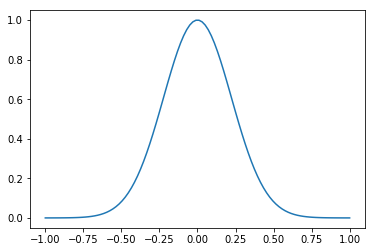### Accessing Numpy Arrays¶

Numpy arrays can be accessed using the bracket operator and are indexed by row and column (by default). If a is an nxn array, then a[i,j] refers to row i and column j.

In :
a = np.zeros((2,2)) # create a 2x2 array of zeros
print(a)
a[0,1] = 33 # change element a[0,1]
print(a)

[[0. 0.]
[0. 0.]]
[[ 0. 33.]
[ 0.  0.]]


On the other hand, a[i] refers to the entire ith row

In :
a = np.zeros((2,2)) # create a 2x2 array of zeros
a = [1,2]
a = [3,4]
print(a)

[[1. 2.]
[3. 4.]]


and a[:,j] refers to the entire jth column.

In :
a = np.zeros((2,2)) # create a 2x2 array of zeros
a[:,0] = [1,3]
a[:,1] = [2,4]
print(a)

[[1. 2.]
[3. 4.]]


The colon in a[:,0] allows you to select a subset of an array. In general is works as: i:j will return the subset array consisting of the elements a[i] to a[j-1]

In :
a = np.arange(1,10)
print(a)
print(a[1:4]) # return the subset array a to a

[1 2 3 4 5 6 7 8 9]
[2 3 4]


If no numbers are provided around the colon (i.e. a[:]), then the entire range is returned

In :
a = np.arange(1,10)
print(a[:])

[1 2 3 4 5 6 7 8 9]


Here's a more complex example of how you can slice through a 4x4 matrix

In :
a = np.zeros((4,4)) # create an array of zeros
print(a)

[[0. 0. 0. 0.]
[0. 0. 0. 0.]
[0. 0. 0. 0.]
[0. 0. 0. 0.]]

In :
# now change row 1, columns 1,2
a[1,1:3] = [1.11,2.22]
print(a)

[[0.   0.   0.   0.  ]
[0.   1.11 2.22 0.  ]
[0.   0.   0.   0.  ]
[0.   0.   0.   0.  ]]

In :
#now change column 3, rows 2,3
a[2:4,3]=[3.33,4.44]
print(a)

[[0.   0.   0.   0.  ]
[0.   1.11 2.22 0.  ]
[0.   0.   0.   3.33]
[0.   0.   0.   4.44]]


### Operations on Numpy Arrays¶

You can operate on arrays using standard mathematical operators as long as the array sizes are consistent with the operation being carried out. All operations are carried out element-wise - that is, applied to each element one at a time.

In :
a = np.arange(0,10) #[0,1,2,...,9]
b = np.arange(10,20) #[10,11,...,19]
a*b

Out:
array([  0,  11,  24,  39,  56,  75,  96, 119, 144, 171])

Mathematical functions provided by numpy will operate on numpy arrays element-wise

In :
a = np.arange(0,10) #[0,1,2,...,9]
np.sqrt(a) # use sqrt provided by numpy

Out:
array([0.        , 1.        , 1.41421356, 1.73205081, 2.        ,
2.23606798, 2.44948974, 2.64575131, 2.82842712, 3.        ])

However, functions provided by other modules, such as the math module, are not guaranteed to work with numpy arrays

In :
import math
a = np.arange(0,10) #[0,1,2,...,9]
math.sqrt(a)

---------------------------------------------------------------------------
TypeError                                 Traceback (most recent call last)
<ipython-input-64-30c328a8cb8e> in <module>()
1 import math
2 a = np.arange(0,10) #[0,1,2,...,9]
----> 3 math.sqrt(a)

TypeError: only size-1 arrays can be converted to Python scalars

In this case, the functions provided by the math module will work on one element at a time, not an entire list, e.g.

math.sqrt(a)


#### Diagonal¶

In :
import numpy as np
A = np.array([[4,-2,1],
[-2,4,-2],
[1,-2,3]])
d = np.diagonal(A) # main diagonal
ud = np.diagonal(A,1) # first upper diagonal
ld = np.diagonal(A,-1) # first lower diagonal
print('main diagonal = ', d)
print('lower diagonal = ', ld)
print('upper diagonal = ', ud)

main diagonal =  [4 4 3]
lower diagonal =  [-2 -2]
upper diagonal =  [-2 -2]


you can also take the trace

In :
tr = np.trace(A)
print(tr)

11


you can create an identity matrix

In :
idm = np.identity(3)
print(idm)

[[1. 0. 0.]
[0. 1. 0.]
[0. 0. 1.]]


#### Dot Product¶

In :
from numpy import *
x = array([7,3])
y = array([2,1])
A = array([[1,2],[3,2]])
B = array([[1,1],[2,2]])

# Dot product
print("dot(x,y) =\n",dot(x,y)) # {x}.{y}
print("dot(A,x) =\n",dot(A,x)) # [A]{x}
print("dot(A,B) =\n",dot(A,B)) # [A][B]

dot(x,y) =
17
dot(A,x) =
[13 27]
dot(A,B) =
[[5 5]
[7 7]]


There's a shorter way of computing dot products. Instead of using dot(x,y), you can use [email protected]

In :
from numpy import *
x = array([7,3])
y = array([2,1])
A = array([[1,2],[3,2]])
B = array([[1,1],[2,2]])

# Dot product
print("[email protected] =\n",x@y) # {x}.{y}
print("[email protected] =\n",A@x) # [A]{x}
print("[email protected] =\n",A@B) # [A][B]

[email protected] =
17
[email protected] =
[13 27]
[email protected] =
[[5 5]
[7 7]]


This depends on your having Python3 installed. Try it, and if it works, then you're likely using python3.

#### Inner Product¶

In :
from numpy import *
x = array([7,3])
y = array([2,1])
A = array([[1,2],[3,2]])
B = array([[1,1],[2,2]])

# Inner product
print("inner(x,y) =\n",inner(x,y))  # {x}.{y}
print("inner(A,x) =\n",inner(A,x))  # [A]{x}
print("inner(A,B) =\n",inner(A,B))  # [A][B_transpose]

inner(x,y) =
17
inner(A,x) =
[13 27]
inner(A,B) =
[[ 3  6]
[ 5 10]]


#### Outer Product¶

In :
from numpy import *
x = array([7,3])
y = array([2,1])
A = array([[1,2],[3,2]])
B = array([[1,1],[2,2]])

# Outer product
print("outer(x,y) =\n",outer(x,y))
print("outer(A,x) =\n",outer(A,x))
print("outer(A,B) =\n",outer(A,B))

outer(x,y) =
[[14  7]
[ 6  3]]
outer(A,x) =
[[ 7  3]
[14  6]
[21  9]
[14  6]]
outer(A,B) =
[[1 1 2 2]
[2 2 4 4]
[3 3 6 6]
[2 2 4 4]]


### Linear Algebra¶

Numpy ships with a linear algebra module named linalg that contains a collection of routines to aid in the solution and manipulation of systems of linear equations.

In :
from numpy import array, dot
from numpy.linalg import inv,solve # import inverse and solve from linalg
A = array([[ 4.0, -2.0,  1.0],
[-2.0,  4.0, -2.0],
[ 1.0, -2.0,  3.0]])
b = array([1.0, 4.0, 2.0])
Ainv = inv(A)

x = solve(A,b) # use built in solve
print(x)

xInv = dot(Ainv,b) # use Ainv . b
print(xInv)

[1.  2.5 2. ]
[1.  2.5 2. ]


# Beauty is in the Eyes of MatPlotLib¶

or how to plot anything in Python!

Matplotlib is the de-facto standard for plotting in Python. Like numpy, matplotlib is a module and supports plotting all sorts of pythonic things.

Here's a simple example plot:

In :
%matplotlib inline
import matplotlib.pyplot as plt # pyplot is the standard plotting library in matplotlib
import numpy as np
x = np.linspace(-1,1,200) # create 200 equally spaced points between -1 and 1
y = np.exp(-x**2/0.01) # gaussian
plt.plot(x,y) # plot y vs x

Out:
[<matplotlib.lines.Line2D at 0x11546ce48>]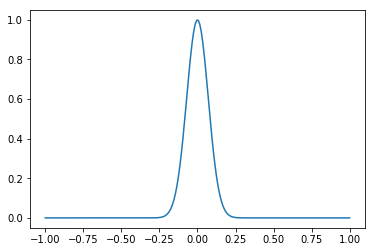you will notice that we imported pyplot from matplotlib. Also note that the statement %matplotlib inline is necessary only when using jupyter notebooks.

you can label axes and add titles to plots:

In :
%matplotlib inline
import matplotlib.pyplot as plt # pyplot is the standard plotting library in matplotlib
import numpy as np
x = np.linspace(-1,1,200) # create 200 equally spaced points between -1 and 1
y = np.exp(-x**2/0.01) # gaussian
plt.title('Gaussian')
plt.xlabel('xlabel', fontsize=18)
plt.ylabel('ylabel', fontsize=16)
plt.plot(x,y) # plot y vs x

Out:
[<matplotlib.lines.Line2D at 0x111b8c898>]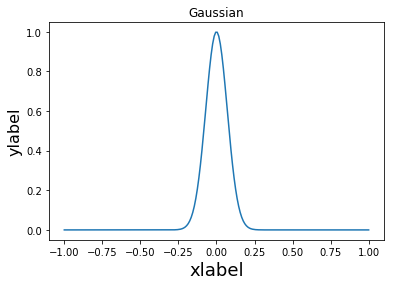you can plot multiple curves

In :
%matplotlib inline
import matplotlib.pyplot as plt # pyplot is the standard plotting library in matplotlib
import numpy as np
x = np.linspace(-1,1,200) # create 200 equally spaced points between -1 and 1
y = np.exp(-x**2/0.01) # gaussian
y2 = np.exp(-x**2/0.1)
plt.title('Gaussians')
plt.xlabel('position', fontsize=18)
plt.ylabel('value', fontsize=16)
plt.plot(x,y,x,y2) # plot y vs x and y2 vs x

Out:
[<matplotlib.lines.Line2D at 0x111baa710>,
<matplotlib.lines.Line2D at 0x11559b1d0>]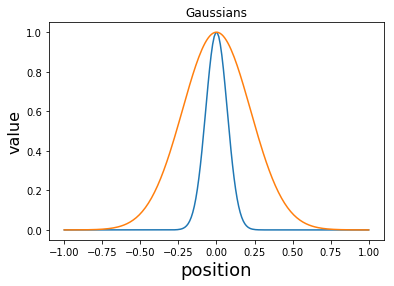you can also add markers and line styles

In :
%matplotlib inline
import matplotlib.pyplot as plt # pyplot is the standard plotting library in matplotlib
import numpy as np
x = np.linspace(-1,1,200) # create 200 equally spaced points between -1 and 1
y = np.exp(-x**2/0.01) # gaussian
y2 = np.exp(-x**2/0.1)
plt.title('Gaussians')
plt.xlabel('position', fontsize=18)
plt.ylabel('value', fontsize=16)
plt.plot(x,y,'r-*',x,y2,'b-.o') # plot y vs x and y2 vs x

Out:
[<matplotlib.lines.Line2D at 0x115569b70>,
<matplotlib.lines.Line2D at 0x1156d0438>]